# 7 Results

View
Selected filters:
• OH.TMM.0005.Core.3Rating

The Calculus I course was developed through the Ohio Department of Higher Education OER Innovation Grant. This work was completed and the course was posted in February 2019. The course is part of the Ohio Transfer Module and is also named TMM005. For more information about credit transfer between Ohio colleges and universities, please visit: www.ohiohighered.org/transfer.Team LeadJim Fowler                                         Ohio State UniversityRita Ralph                                         Columbus State Community CollegeContent ContributorsNela Lakos                                       Ohio State UniversityBart Snapp                                       Ohio State UniversityJames Talamo                                  Ohio State UniversityXiang Yan                                         Edison State Community CollegeLibrarianDaniel Dotson                                    Ohio State University                     Review TeamThomas Needham                             Ohio State UniversityCarl Stitz                                            Lakeland Community CollegeSara Rollo                                          North Central State College

Subject:
Mathematics
Calculus
Material Type:
Full Course
Provider:
Ohio Open Ed Collaborative
09/26/2018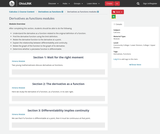Conditional Remix & Share Permitted
CC BY-NC
Rating

After completing this section, students should be able to do the following.Understand the derivative as a function related to the original definition of a function.Find the derivative function using the limit definition.Relate the derivative function to the derivative at a point.Explain the relationship between differentiability and continuity.Relate the graph of the function to the graph of its derivative.Determine whether a piecewise function is differentiable.

Subject:
Calculus
Material Type:
Module
Provider:
Ohio Open Ed Collaborative
06/28/2019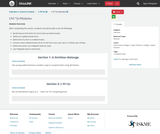Conditional Remix & Share Permitted
CC BY-NC
Rating

After completing this section, students should be able to do the following.Recall how to find limits for forms that are determinate.Define an indeterminate form.Determine if a form is indeterminate.Convert some indeterminate forms to the form zero over zero or infinity over infinity.Determine when can l’Hôpital’s Rule be used.Use l’Hôpital’s Rule to find limits.

Subject:
Calculus
Material Type:
Module
Provider:
Ohio Open Ed Collaborative
07/03/2019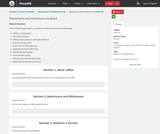Conditional Remix & Share Permitted
CC BY-NC
Rating

After completing this section, students should be able to do the following.Define a critical point.Find critical points.Define local maximum and local minimum.Classify critical points.State the First Derivative Test.Apply the First Derivative Test.Define inflection points.Find inflection points.State the Second Derivative Test.Apply the Second Derivative Test.

Subject:
Calculus
Material Type:
Module
Provider:
Ohio Open Ed Collaborative
07/02/2019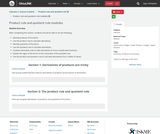Conditional Remix & Share Permitted
CC BY-NC
Rating

After completing this section, students should be able to do the following.Identify products of functions.Use the product rule to calculate derivatives.Identify quotients of functions.Use the quotient rule to calculate derivatives.Combine derivative rules to take derivatives of more complicated functions.Explain the signs of the terms in the numerator of the quotient rule.Use the product and quotient rule to calculate derivatives from a table of values.

Subject:
Calculus
Material Type:
Module
Provider:
Ohio Open Ed Collaborative
07/02/2019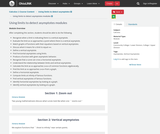Conditional Remix & Share Permitted
CC BY-NC
Rating

After completing this section, students should be able to do the following.Recognize when a limit is indicating there is a vertical asymptote.Evaluate the limit as xx approaches a point where there is a vertical asymptote.Match graphs of functions with their equations based on vertical asymptotes.Discuss what it means for a limit to equal ∞∞.Define a vertical asymptote.Find horizontal asymptotes using limits.Produce a function with given asymptotic behavior.Recognize that a curve can cross a horizontal asymptote.Understand the relationship between limits and vertical asymptotes.Calculate the limit as xx approaches ±∞±∞ of common functions algebraically.Find the limit as xx approaches ±∞±∞ from a graph.Define a horizontal asymptote.Compute limits at infinity of famous functions.Find vertical asymptotes of famous functions.Identify horizontal asymptotes by looking at a graph.Identify vertical asymptotes by looking at a graph.

Subject:
Calculus
Material Type:
Module
Provider:
Ohio Open Ed Collaborative
06/28/2019Unrestricted Use
CC BY
Rating

This is a Calculus I interactive textbook with modules curated and created on The Ohio State University's Ximera platform.

Subject:
Mathematics
Calculus
Material Type:
Textbook
Provider:
Columbus State Community College
Author:
Kevin James
Lee Wayand
Rita Ralph
The Ximera Project
Tzy-Yi "Alan" Yang